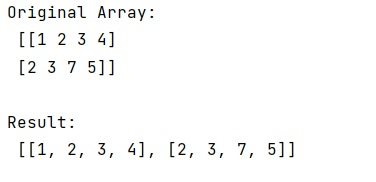# Convert 2d numpy array into list of lists

Learn, how to convert 2d numpy array into list of lists in Python?
Submitted by Pranit Sharma, on January 06, 2023

NumPy is an abbreviated form of Numerical Python. It is used for different types of scientific operations in python. Numpy is a vast library in python which is used for almost every kind of scientific or mathematical operation. It is itself an array which is a collection of various methods and functions for processing the arrays.

## Converting a 2d numpy array into list of lists

We know how to convert a list of lists into a two-dimensional array but suppose we are already given a two-dimensional array and we need to convert it into a list of lists.

A list is a collection of heterogeneous elements and it is mutable. Elements inside the list are encapsulated in square brackets. A list is considered a series or collection and we can perform operations like insert, update, and delete with its elements.

For this purpose, we can simply cast the matrix to list with matrix.tolist().

Let us understand with the help of an example,

## Python code to convert 2d numpy array into list of lists

```# Import numpy
import numpy as np

# Creating an array
arr = np.array([[1,2,3,4],[2,3,7,5]])

# Display original array
print("Original Array:\n",arr,"\n")

# Converting matrix into list
res = arr.tolist()

# Display result
print("Result:\n",res,"\n")
```

Output: